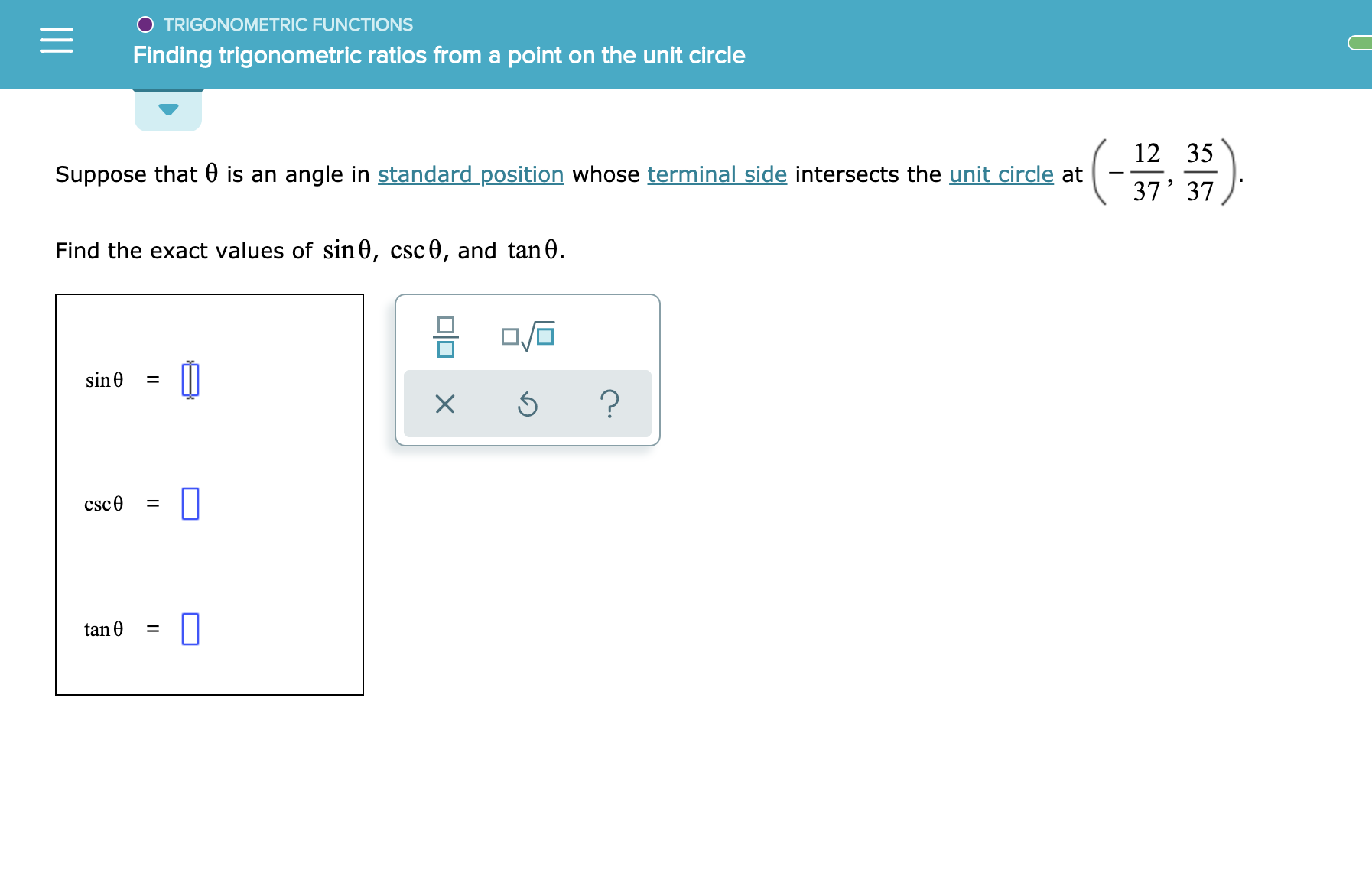# TRIGONOMETRIC FUNCTIONSFinding trigonometric ratios from a point on the unit circle12 35Suppose that 0 is an angle in standard position whose terminal side intersects the unit circle at37' 37Find the exact values of sin0, csc0, and tan0.sin 0?csc0tan 0L

Question
24 views

See attachmenthelp_outlineImage TranscriptioncloseTRIGONOMETRIC FUNCTIONS Finding trigonometric ratios from a point on the unit circle 12 35 Suppose that 0 is an angle in standard position whose terminal side intersects the unit circle at 37' 37 Find the exact values of sin0, csc0, and tan0. sin 0 ? csc0 tan 0 L fullscreen
check_circle

Step 1

If θ is an angle in standard position whose terminal sides intersects the unit circle at (-12/37,35/37).

Step 2

Find Sin θ, Csc θ, Tan θ

Step 3

Given a right triangl...

### Want to see the full answer?

See Solution

#### Want to see this answer and more?

Solutions are written by subject experts who are available 24/7. Questions are typically answered within 1 hour.*

See Solution
*Response times may vary by subject and question.
Tagged in

### Other